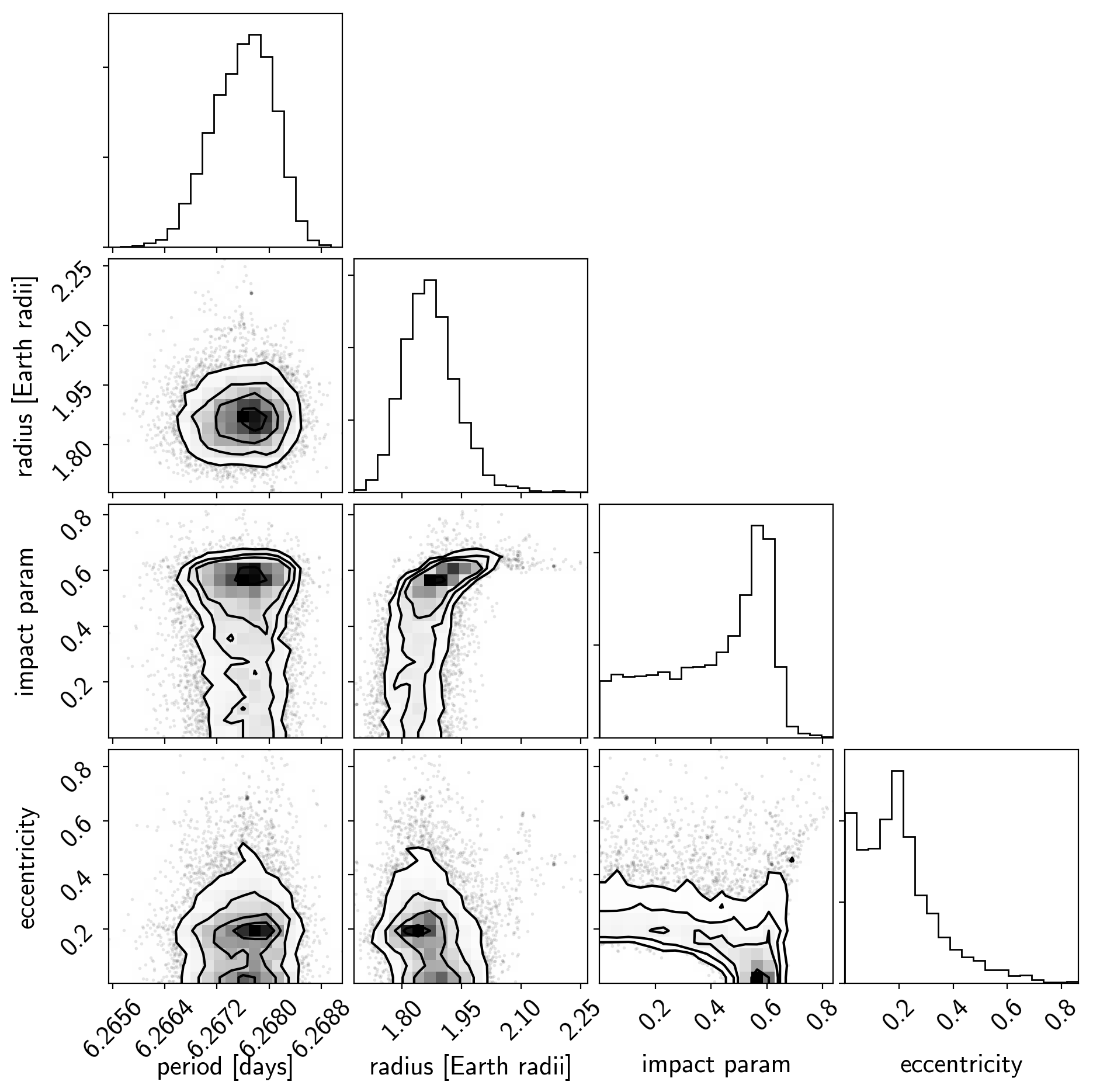Note

This tutorial was generated from an IPython notebook that can be downloaded here.

# Fitting TESS data¶

In this tutorial, we will reproduce the fits to the transiting planet in the Pi Mensae system discovered by Huang et al. (2018). The data processing and model are similar to the Case study: K2-24, putting it all together tutorial, but with a few extra bits like aperture selection and de-trending.

import numpy as np
from astropy.io import fits
import matplotlib.pyplot as plt

tpf_url = "https://archive.stsci.edu/missions/tess/tid/s0001/0000/0002/6113/6679/tess2018206045859-s0001-0000000261136679-0120-s_tp.fits"
with fits.open(tpf_url) as hdus:
tpf = hdus.data

texp = tpf_hdr["FRAMETIM"] * tpf_hdr["NUM_FRM"]
texp /= 60.0 * 60.0 * 24.0
time = tpf["TIME"]
flux = tpf["FLUX"]
m = np.any(np.isfinite(flux), axis=(1, 2)) & (tpf["QUALITY"] == 0)
ref_time = 0.5 * (np.min(time[m])+np.max(time[m]))
time = np.ascontiguousarray(time[m] - ref_time, dtype=np.float64)
flux = np.ascontiguousarray(flux[m], dtype=np.float64)

mean_img = np.median(flux, axis=0)
plt.imshow(mean_img.T, cmap="gray_r")
plt.title("TESS image of Pi Men")
plt.xticks([])
plt.yticks([]);## Aperture selection¶

Next, we’ll select an aperture using a hacky method that tries to minimizes the windowed scatter in the lightcurve (something like the CDPP).

from scipy.signal import savgol_filter

# Sort the pixels by median brightness
order = np.argsort(mean_img.flatten())[::-1]

# A function to estimate the windowed scatter in a lightcurve
smooth = savgol_filter(f, 1001, polyorder=5)
return 1e6 * np.sqrt(np.median((f / smooth - 1)**2))

# Loop over pixels ordered by brightness and add them one-by-one
# to the aperture
for i in range(10, 100):
msk = np.zeros_like(mean_img, dtype=bool)
msk[np.unravel_index(order[:i], mean_img.shape)] = True
scatters.append(scatter)

# Choose the aperture that minimizes the scatter

# Plot the selected aperture
plt.imshow(mean_img.T, cmap="gray_r")
plt.title("selected aperture")
plt.xticks([])
plt.yticks([]);This aperture produces the following light curve:

plt.figure(figsize=(10, 5))
sap_flux = (sap_flux / np.median(sap_flux) - 1) * 1e3
plt.plot(time, sap_flux, "k")
plt.xlabel("time [days]")
plt.ylabel("relative flux [ppt]")
plt.title("raw light curve")
plt.xlim(time.min(), time.max());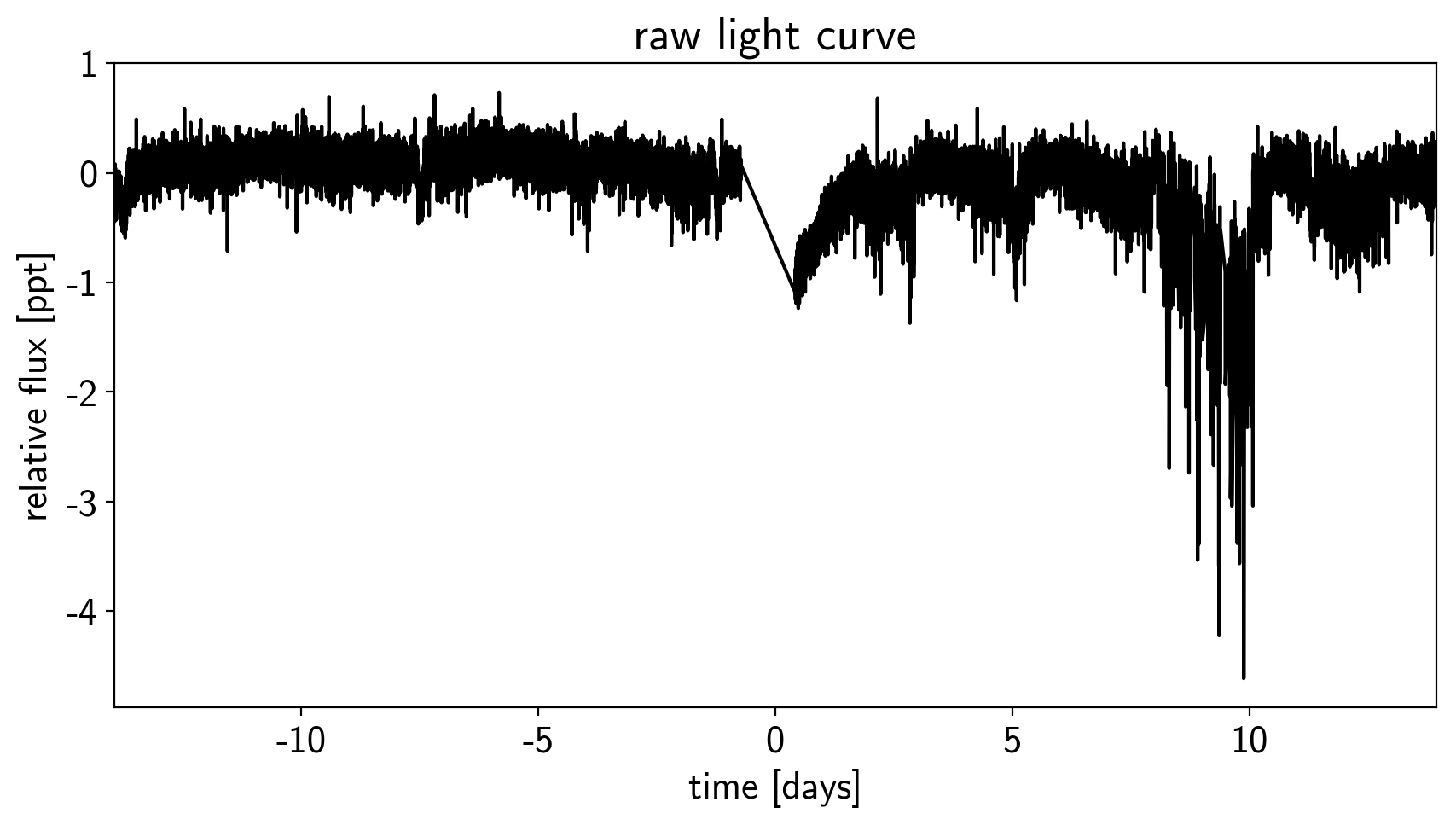## The transit model in PyMC3¶

The transit model, initialization, and sampling are all nearly the same as the one in Case study: K2-24, putting it all together, but we’ll use a more informative prior on eccentricity.

import exoplanet as xo
import pymc3 as pm
import theano.tensor as tt

with pm.Model() as model:

# Parameters for the stellar properties
mean = pm.Normal("mean", mu=0.0, sd=10.0)

# Stellar parameters from Huang et al (2018)
M_star_huang = 1.094, 0.039
R_star_huang = 1.10, 0.023
m_star = pm.Normal("m_star", mu=M_star_huang, sd=M_star_huang)
r_star = pm.Normal("r_star", mu=R_star_huang, sd=R_star_huang)

# Prior to require physical parameters
pm.Potential("m_star_prior", tt.switch(m_star > 0, 0, -np.inf))
pm.Potential("r_star_prior", tt.switch(r_star > 0, 0, -np.inf))

# Orbital parameters for the planets
logP = pm.Normal("logP", mu=np.log(bls_period), sd=1)
t0 = pm.Normal("t0", mu=bls_t0, sd=1)
testval_r=np.sqrt(1e-3)*np.sqrt(bls_depth),
testval_b=0.5)

# This is the eccentricity prior from Kipping (2013):
# https://arxiv.org/abs/1306.4982
ecc = pm.Beta("ecc", alpha=0.867, beta=3.03, testval=0.1)
omega = xo.distributions.Angle("omega")

# Log-uniform prior on ror
pm.Potential("ror_prior", -tt.log(ror))

# Transit jitter & GP parameters
logw0 = pm.Normal("logw0", mu=np.log(2*np.pi/10), sd=10)

# Tracking planet parameters
period = pm.Deterministic("period", tt.exp(logP))
r_pl = pm.Deterministic("r_pl", r_star * ror)

# Orbit model
orbit = xo.orbits.KeplerianOrbit(
r_star=r_star, m_star=m_star,
period=period, t0=t0, b=b,
ecc=ecc, omega=omega)

# Compute the model light curve using starry
light_curves = xo.StarryLightCurve(u_star).get_light_curve(
light_curve = pm.math.sum(light_curves, axis=-1) + mean
pm.Deterministic("light_curves", light_curves)

# GP model for the light curve
kernel = xo.gp.terms.SHOTerm(log_S0=logS0, log_w0=logw0, Q=1/np.sqrt(2))
pm.Deterministic("gp_pred", gp.predict())

# Fit for the maximum a posteriori parameters, I've found that I can get
# a better solution by trying different combinations of parameters in turn
if start is None:
start = model.test_point
map_soln = pm.find_MAP(start=start, vars=[logs2, logS0, logw0])
map_soln = pm.find_MAP(start=map_soln, vars=[model.rb])
map_soln = pm.find_MAP(start=map_soln)

return model, map_soln

model0, map_soln0 = build_model()

logp = 12,994, ||grad|| = 315.08: 100%|██████████| 29/29 [00:00<00:00, 85.92it/s]
logp = 13,001, ||grad|| = 47.911: 100%|██████████| 10/10 [00:00<00:00, 94.58it/s]
logp = 13,020, ||grad|| = 258.8: 100%|██████████| 14/14 [00:00<00:00, 83.02it/s]


Here’s how we plot the initial light curve model:

def plot_light_curve(soln, mask=None):

fig, axes = plt.subplots(3, 1, figsize=(10, 7), sharex=True)

ax = axes
gp_mod = soln["gp_pred"] + soln["mean"]
ax.legend(fontsize=10)
ax.set_ylabel("relative flux [ppt]")

ax = axes
for i, l in enumerate("b"):
mod = soln["light_curves"][:, i]
ax.legend(fontsize=10, loc=3)
ax.set_ylabel("de-trended flux [ppt]")

ax = axes
mod = gp_mod + np.sum(soln["light_curves"], axis=-1)
ax.axhline(0, color="#aaaaaa", lw=1)
ax.set_ylabel("residuals [ppt]")
ax.set_xlabel("time [days]")

return fig

plot_light_curve(map_soln0);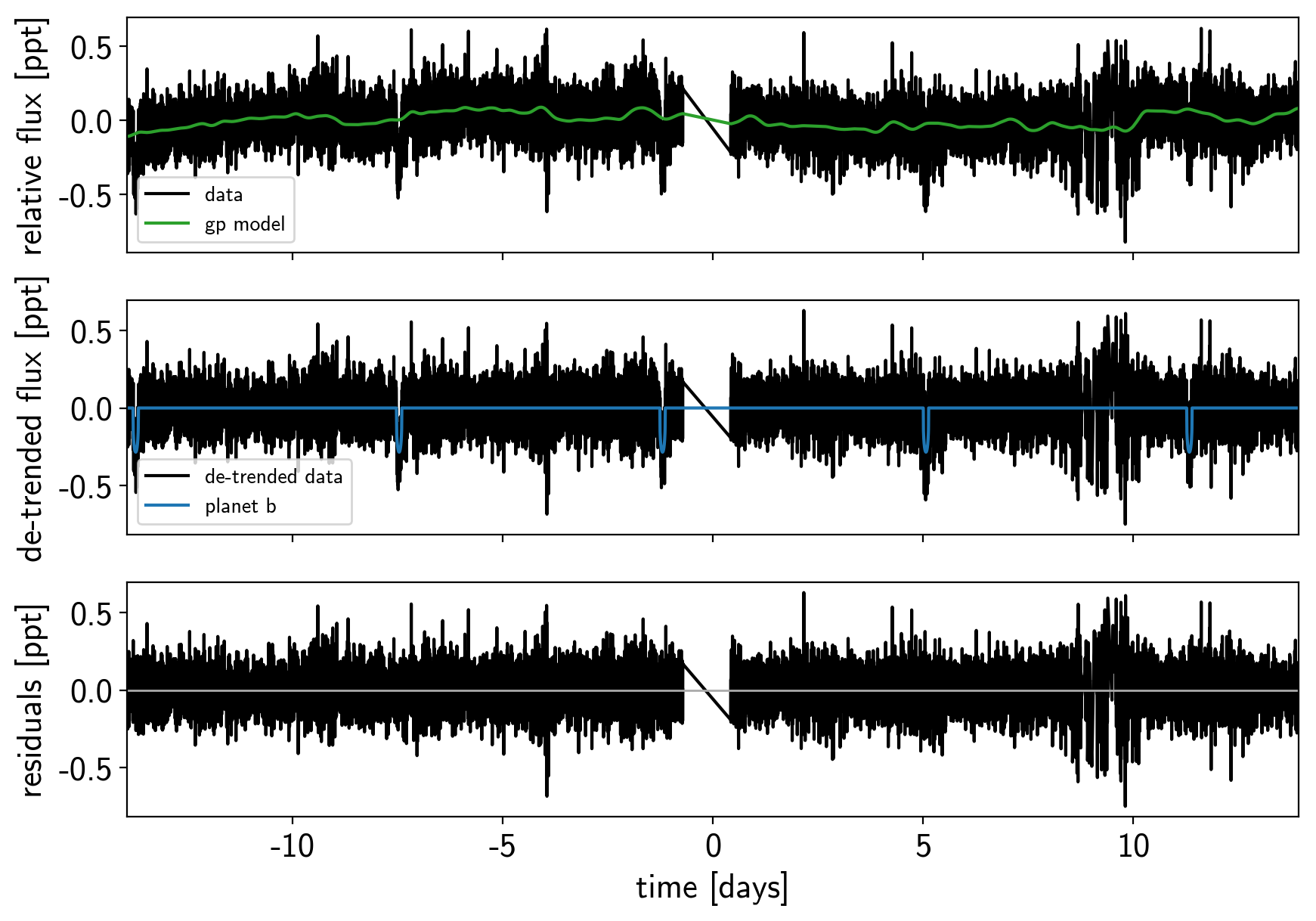As in the Case study: K2-24, putting it all together tutorial, we can do some sigma clipping to remove significant outliers.

mod = map_soln0["gp_pred"] + map_soln0["mean"] + np.sum(map_soln0["light_curves"], axis=-1)
resid = y - mod
rms = np.sqrt(np.median(resid**2))
mask = np.abs(resid) < 5 * rms

plt.figure(figsize=(10, 5))
plt.plot(x, resid, "k", label="data")
plt.axhline(0, color="#aaaaaa", lw=1)
plt.ylabel("residuals [ppt]")
plt.xlabel("time [days]")
plt.legend(fontsize=12, loc=3)
plt.xlim(x.min(), x.max());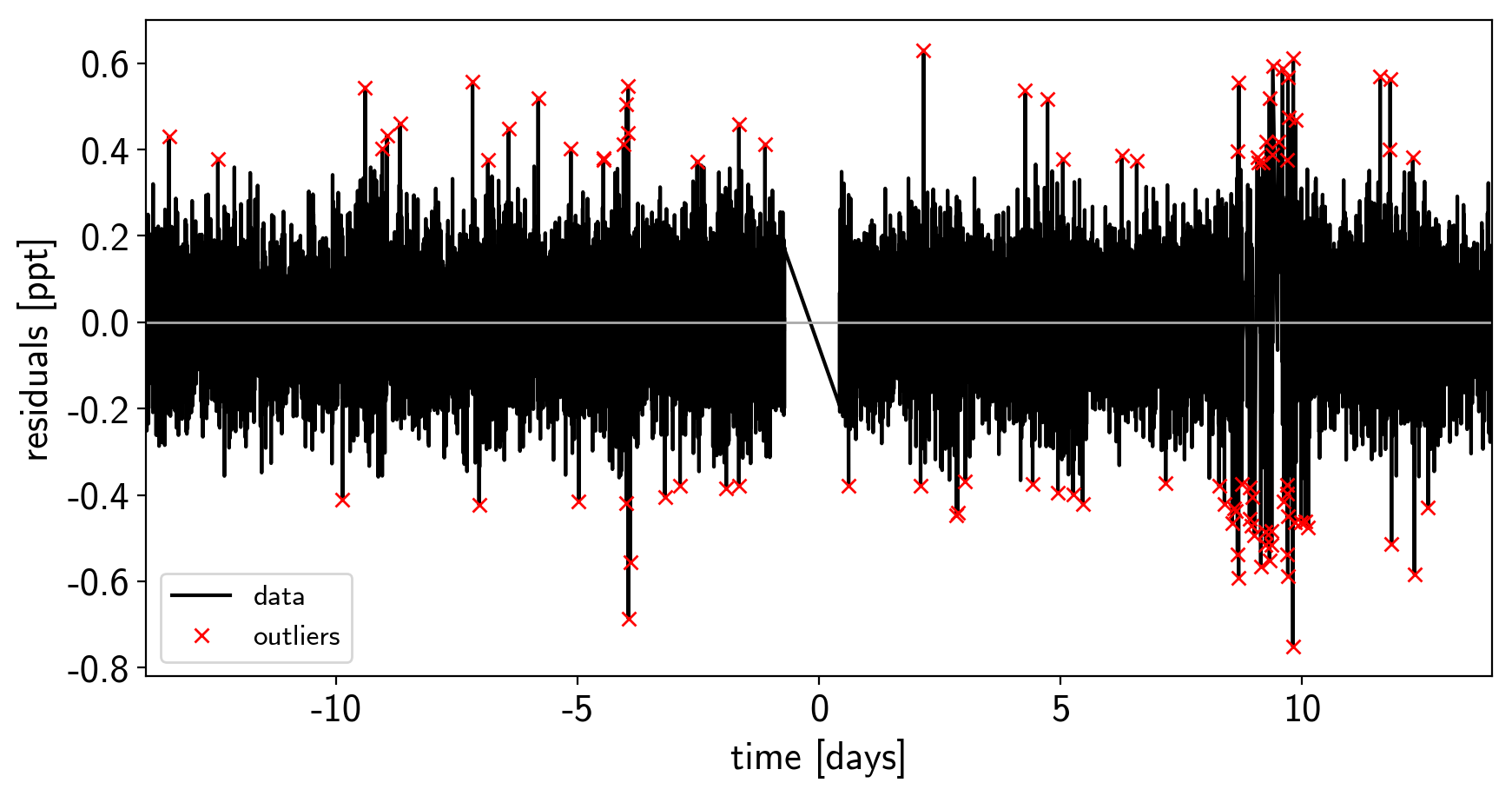And then we re-build the model using the data without outliers.

model, map_soln = build_model(mask, map_soln0)

logp = 13,724, ||grad|| = 1.7255: 100%|██████████| 17/17 [00:00<00:00, 89.34it/s]
logp = 13,724, ||grad|| = 11.222: 100%|██████████| 8/8 [00:00<00:00, 109.98it/s]
logp = 13,724, ||grad|| = 48.236: 100%|██████████| 12/12 [00:00<00:00, 85.13it/s]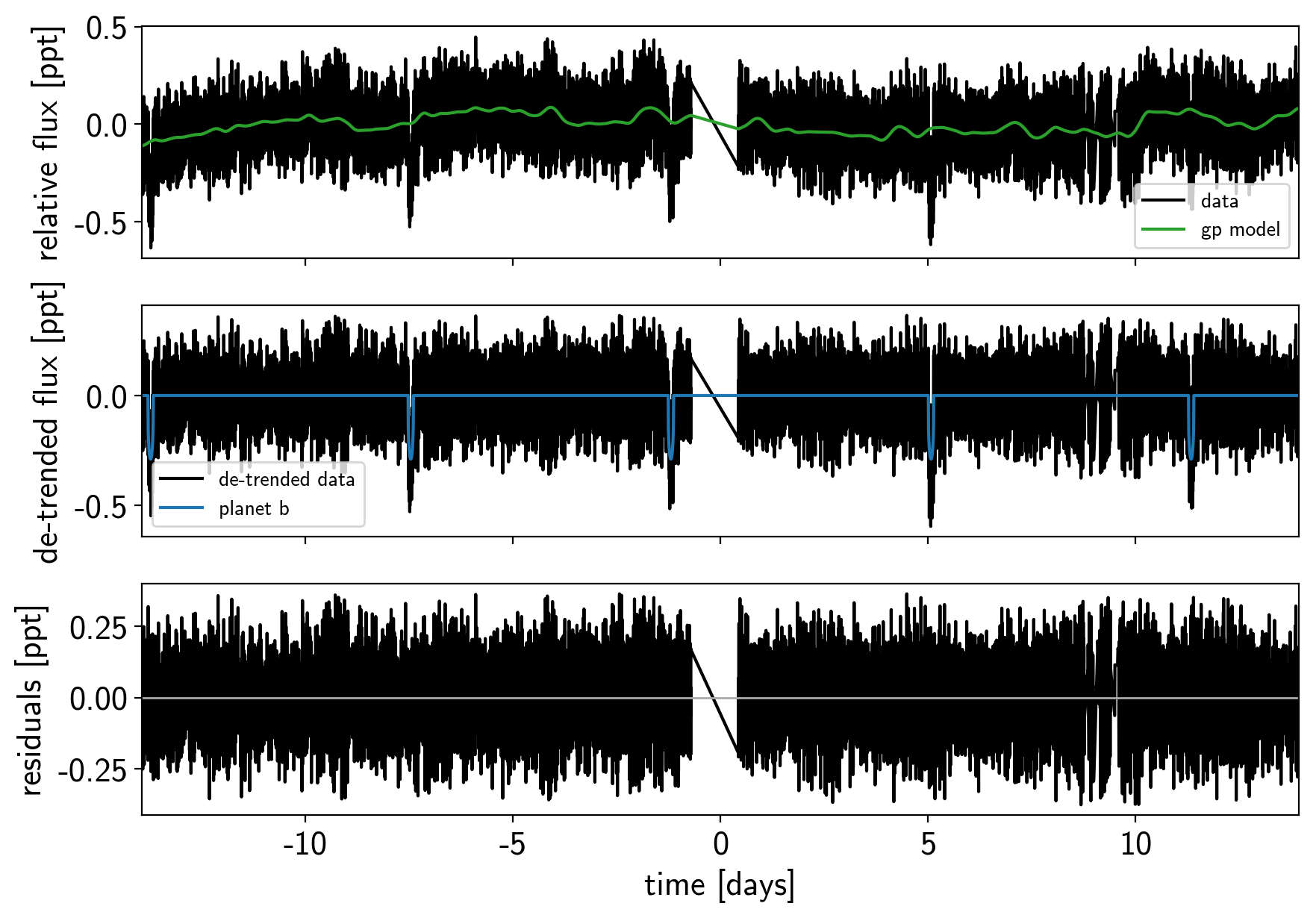Now that we have the model, we can sample it using a exoplanet.PyMC3Sampler:

np.random.seed(42)
sampler = xo.PyMC3Sampler(window=100, start=200, finish=200)
with model:
burnin = sampler.tune(tune=3000, start=map_soln, step_kwargs=dict(target_accept=0.9))

Sampling 4 chains: 100%|██████████| 808/808 [06:51<00:00,  3.23s/draws]
Sampling 4 chains: 100%|██████████| 408/408 [03:22<00:00,  2.02s/draws]
Sampling 4 chains: 100%|██████████| 808/808 [06:32<00:00,  1.80s/draws]
Sampling 4 chains: 100%|██████████| 1608/1608 [15:13<00:00,  1.55s/draws]
Sampling 4 chains: 100%|██████████| 8408/8408 [1:13:25<00:00,  2.17s/draws]

with model:
trace = sampler.sample(draws=2000)

Multiprocess sampling (4 chains in 4 jobs)
NUTS: [logw0, logS0, logs2, omega, ecc, rb, t0, logP, r_star, m_star, u_star, mean]
Sampling 4 chains: 100%|██████████| 8800/8800 [1:55:09<00:00,  3.46s/draws]
There were 3 divergences after tuning. Increase target_accept or reparameterize.
There were 46 divergences after tuning. Increase target_accept or reparameterize.
There were 11 divergences after tuning. Increase target_accept or reparameterize.
There were 17 divergences after tuning. Increase target_accept or reparameterize.
The number of effective samples is smaller than 25% for some parameters.

pm.summary(trace, varnames=["logw0", "logS0", "logs2", "omega", "ecc", "r", "b", "t0", "logP", "r_star", "m_star", "u_star", "mean"])

mean sd mc_error hpd_2.5 hpd_97.5 n_eff Rhat
logw0 1.169933 0.134382 1.710553e-03 9.057975e-01 1.426173 5380.444081 1.000417
logS0 -6.794914 0.340207 4.232829e-03 -7.430404e+00 -6.112015 5193.502252 0.999900
logs2 -4.383342 0.010547 1.218625e-04 -4.403523e+00 -4.362676 6670.767506 0.999829
omega 0.741572 1.685123 3.409555e-02 -2.847012e+00 3.139181 2228.642932 1.000115
ecc 0.199290 0.143478 2.788730e-03 7.505986e-07 0.483658 2205.279438 1.001101
r__0 0.015617 0.000447 1.265344e-05 1.481963e-02 0.016442 1136.195700 1.001984
b__0 0.407585 0.192050 4.839018e-03 3.120207e-02 0.649304 1444.097405 1.000071
t0 -1.197506 0.000668 1.236398e-05 -1.198828e+00 -1.196184 2557.054774 1.000829
logP 1.835392 0.000075 9.508086e-07 1.835247e+00 1.835527 5164.781290 0.999993
r_star 1.098558 0.023103 2.872805e-04 1.054516e+00 1.144394 6021.620225 1.000639
m_star 1.095636 0.038200 4.404067e-04 1.022309e+00 1.170718 7402.759081 0.999830
u_star__0 0.195088 0.163378 2.450729e-03 1.297818e-04 0.523474 4238.611904 1.000690
u_star__1 0.523479 0.263035 4.398927e-03 -1.104263e-02 0.955436 3107.721682 1.000902
mean -0.001184 0.009222 1.212084e-04 -1.939158e-02 0.016804 6015.058717 1.000076

## Results¶

After sampling, we can make the usual plots. First, let’s look at the folded light curve plot:

# Compute the GP prediction
gp_mod = np.median(trace["gp_pred"] + trace["mean"][:, None], axis=0)

# Get the posterior median orbital parameters
p = np.median(trace["period"])
t0 = np.median(trace["t0"])

# Plot the folded data
x_fold = (x[mask] - t0 + 0.5*p) % p - 0.5*p
plt.plot(x_fold, y[mask] - gp_mod, ".k", label="data", zorder=-1000)

# Overplot the phase binned light curve
bins = np.linspace(-0.41, 0.41, 50)
denom, _ = np.histogram(x_fold, bins)
num, _ = np.histogram(x_fold, bins, weights=y[mask])
denom[num == 0] = 1.0
plt.plot(0.5*(bins[1:] + bins[:-1]), num / denom, "o", color="C2",
label="binned")

# Plot the folded model
inds = np.argsort(x_fold)
inds = inds[np.abs(x_fold)[inds] < 0.3]
pred = trace["light_curves"][:, inds, 0]
pred = np.percentile(pred, [16, 50, 84], axis=0)
plt.plot(x_fold[inds], pred, color="C1", label="model")
art = plt.fill_between(x_fold[inds], pred, pred, color="C1", alpha=0.5,
zorder=1000)
art.set_edgecolor("none")

# Annotate the plot with the planet's period
txt = "period = {0:.5f} +/- {1:.5f} d".format(
np.mean(trace["period"]), np.std(trace["period"]))
plt.annotate(txt, (0, 0), xycoords="axes fraction",
xytext=(5, 5), textcoords="offset points",
ha="left", va="bottom", fontsize=12)

plt.legend(fontsize=10, loc=4)
plt.xlim(-0.5*p, 0.5*p)
plt.xlabel("time since transit [days]")
plt.ylabel("de-trended flux")
plt.xlim(-0.15, 0.15);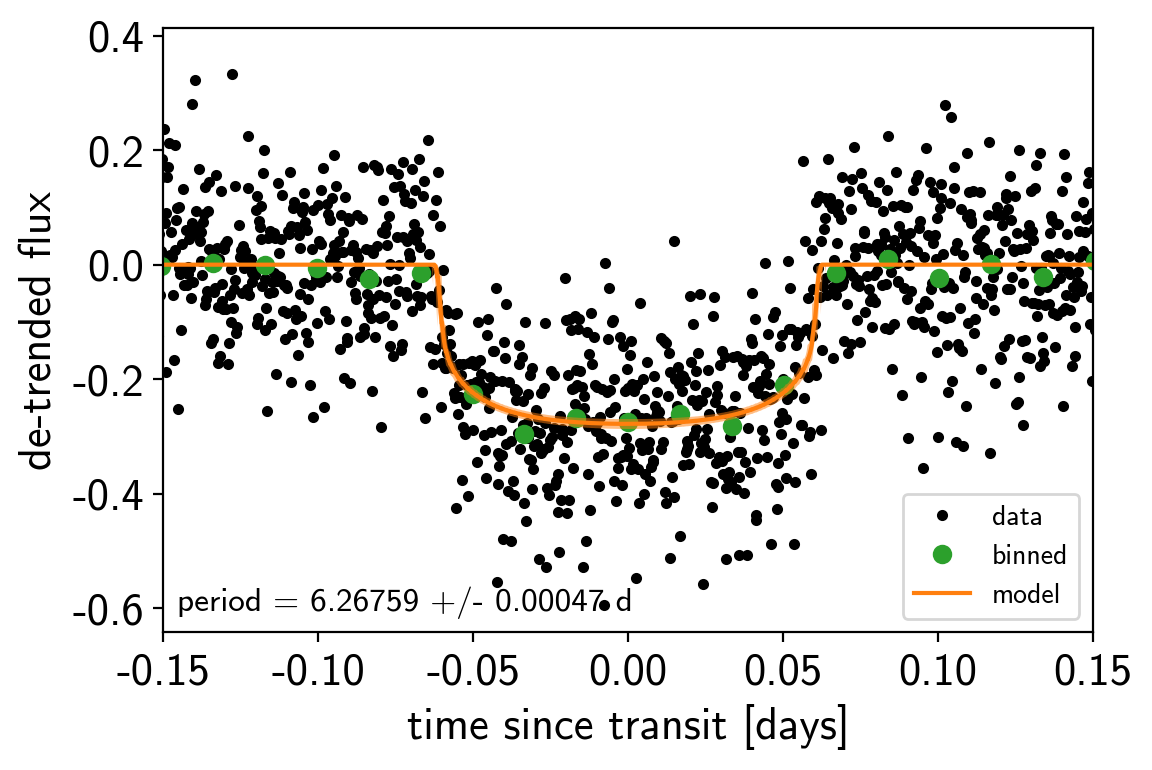And a corner plot of some of the key parameters:

import corner
import astropy.units as u
varnames = ["period", "b", "ecc", "r_pl"]
samples = pm.trace_to_dataframe(trace, varnames=varnames)